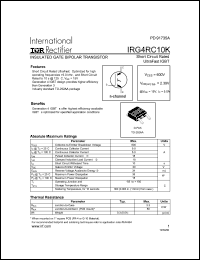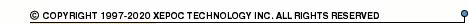More than4 307 195 components listedIRG4RC10 series datasheets. Manufacturer: International Rectifier.

 IRG4RC10K Insulated gate bipolar transistor. VCES = 600V, VCE(on)typ. = 2.39V @ VGE = 15V, IC = 5.0A in 3-pin D-PAK package. Operational temperature range from -55°C to 150°C. Datasheet*) IRG4RC10K Insulated gate bipolar transistor. VCES = 600V, VCE(on)typ. = 2.39V @ VGE = 15V, IC = 5.0A in 3-pin TO-252AA package. Operational temperature range from -55°C to 150°C. Datasheet*) IRG4RC10KD Insulated gate bipolar transistor with ultrafast soft recovery diode. VCES = 600V, VCE(on)typ. = 2.39V @ VGE = 15V, IC = 5.0A in 3-pin TO-252AA package. Operational temperature range from -55°C to 150°C. Datasheet*) IRG4RC10KD Insulated gate bipolar transistor with ultrafast soft recovery diode. VCES = 600V, VCE(on)typ. = 2.39V @ VGE = 15V, IC = 5.0A in 3-pin D-PAK package. Operational temperature range from -55°C to 150°C. Datasheet*) IRG4RC10SD Insulated gate bipolar transistor with ultrafast soft recovery diode. VCES = 600V, VCE(on)typ. = 1.10V @ VGE = 15V, IC = 2.0A in 3-pin D-PAK package. Operational temperature range from -55°C to 150°C. Datasheet*) IRG4RC10SD Insulated gate bipolar transistor with ultrafast soft recovery diode. VCES = 600V, VCE(on)typ. = 1.10V @ VGE = 15V, IC = 2.0A in 3-pin TO-252AA package. Operational temperature range from -55°C to 150°C. Datasheet*) IRG4RC10S Insulated gate bipolar transistor. VCES = 600V, VCE(on)typ. = 1.10V @ VGE = 15V, IC = 2.0A in 3-pin TO-252AA package. Operational temperature range from -55°C to 150°C. Datasheet*) IRG4RC10S Insulated gate bipolar transistor. VCES = 600V, VCE(on)typ. = 1.10V @ VGE = 15V, IC = 2.0A in 3-pin D-PAK package. Operational temperature range from -55°C to 150°C. Datasheet*) IRG4RC10U Insulated gate bipolar transistor. VCES = 600V, VCE(on)typ. = 2.15V @ VGE = 15V, IC = 5.0A in 3-pin D-PAK package. Operational temperature range from -55°C to 150°C. Datasheet*) IRG4RC10U Insulated gate bipolar transistor. VCES = 600V, VCE(on)typ. = 2.15V @ VGE = 15V, IC = 5.0A in 3-pin TO-252AA package. Operational temperature range from -55°C to 150°C. Datasheet*) IRG4RC10UD Insulated gate bipolar transistor with ultrafast soft recovery diode. VCES = 600V, VCE(on)typ. = 2.15V @ VGE = 15V, IC = 5.0A, tf(typ) = 140ns. in 3-pin TO-252AA package. Operational temperature range from -55°C to 150°C. Datasheet*) IRG4RC10UD Insulated gate bipolar transistor with ultrafast soft recovery diode. VCES = 600V, VCE(on)typ. = 2.15V @ VGE = 15V, IC = 5.0A, tf(typ) = 140ns. in 3-pin D-PAK package. Operational temperature range from -55°C to 150°C. Datasheet*) IRG4RC10 INSULATED GATE BIPOLAR TRANSISTOR(Vces=600V, Vce(on)typ.=2.39V, @Vge=15V, Ic=5.0A) Datasheet*)